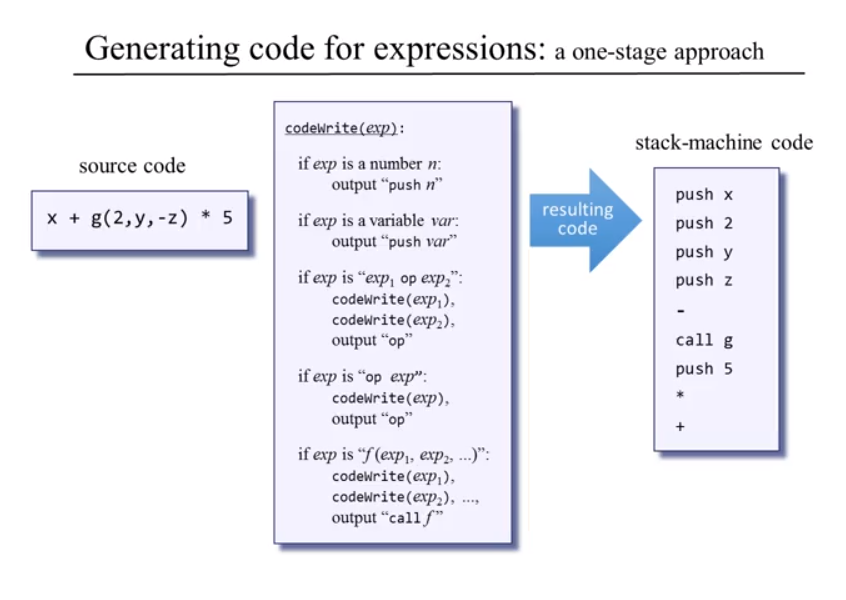# codeWriter(exp)ClassicListThreaded3 messagesOpen this post in threaded view
|

## codeWriter(exp)

 This post was updated on .From what I understand from the lecture, expression is 'term (op term)*' ('*' means it's 0 or more.) So if I follow this rule, there is no condition that matches this case( x + g(2,y,-z) * 5 ). The source code is basically 'term op term op term'. Even If I split up the expression to two parts, 1. x + g(2,y,-z) 2. (result of 1) * 5 The resulting code makes no sense because 1. exp1( x ) op( + ) exp2( g(2,y,-z) ) will generate push x push 2 push y push z push - call g push + 2. exp1( result of 1 ) op( * ) exp2 ( 5 ) will generate push x push 2 push y push z push - call g push + push 5 push * Because Jack language has no operator priority. Should I implement my own version of codeWrite(exp)? Also, I have no idea how should I prioritize expressions inside of parenthesis. edit 1: Is it ok to let the compiler calculate integer-only-expressions? Like, 'do Output.printInt(1 + (2 * 3));' can be simplified during compile time into 'do Output.printInt(7)' edit 2 : And... Is it really possible to reuse Project 10's Compilation Engine?? For example, A variable declaration actually doesn't have to be placed in the first line of the function in Jack language. So the safest time to count local arguments would be right before the 'return' statement. Then somehow I should delay the rest of .vm writing for 'function name nLocals' ... Or Is it safe to assume that var dec always will be in the first line of the function.
 Administrator there is no condition that matches this case( x + g(2,y,-z) * 5 ). Here is the parse tree that matches the expression. ```expression: x + g(2,y,-z) * 5 | term: x | op: + | term: g(2,y,-z) | | subroutineCall: g(2,y,-z) | | | subroutineName: g | | | expressionList: (2,y,-z) | | | | expression: 2 | | | | | term: 2 | | | | expression: y | | | | | term: y | | | | expression: -z | | | | | term: -z | | | | | | unaryOp: - | | | | | | term z | op: * | term: 5 ```Note: "push -" should be the "neg" VM command. "push +" should be the "add". "push *" should be "call Math.multiply 2". Should I implement my own version of codeWrite(exp)? This is the beautiful part of the recursive descent compiler design: You do not need to write codewrite(exp), just call compileExpression() and the appropriate code will be written when it recursively calls compileTerm(). Is it ok to let the compiler calculate integer-only-expressions? Yes, that is OK, but it is a lot of extra work! --Mark